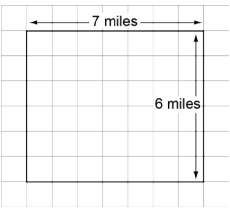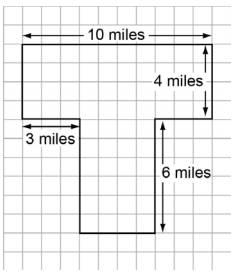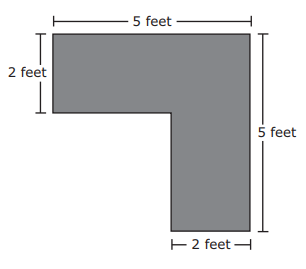# MAFS.3.MD.3.7

Relate area to the operations of multiplication and addition.
1. Find the area of a rectangle with whole-number side lengths by tiling it, and show that the area is the same as would be found by multiplying the side lengths.
2. Multiply side lengths to find areas of rectangles with whole-number side lengths in the context of solving real world and mathematical problems, and represent whole-number products as rectangular areas in mathematical reasoning.
3. Use tiling to show in a concrete case that the area of a rectangle with whole-number side lengths a and b + c is the sum of a × b and a × c. Use area models to represent the distributive property in mathematical reasoning.
4. Recognize area as additive. Find areas of rectilinear figures by decomposing them into non-overlapping rectangles and adding the areas of the non-overlapping parts, applying this technique to solve real world problems.

### Clarifications

Examples of Opportunities for In-Depth Focus

Area is a major concept within measurement, and area models must function as a support for multiplicative reasoning in grade 3 and beyond.
General Information
Subject Area: Mathematics
Domain-Subdomain: Measurement and Data
Cluster: Level 3: Strategic Thinking & Complex Reasoning
Cluster: Geometric measurement: understand concepts of area and relate area to multiplication and to addition. (Major Cluster) -

Clusters should not be sorted from Major to Supporting and then taught in that order. To do so would strip the coherence of the mathematical ideas and miss the opportunity to enhance the major work of the grade with the supporting clusters.

Date of Last Rating: 02/14
Status: State Board Approved
Assessed: Yes
Test Item Specifications

• Assessment Limits :
Figures are limited to rectangles and shapes that can be decomposed into rectangles. Dimensions of figures are limited to whole numbers. All values in items may not exceed whole number multiplication facts of 10 x 10.
• Calculator :
No
• Context :

Allowable

Sample Test Items (3)
• Test Item #: Sample Item 1
• Question:

A park is in the shape of the rectangle shown.What is the area, in square miles, of the park?

• Difficulty: N/A
• Type: EE: Equation Editor

• Test Item #: Sample Item 2
• Question:

A park is shown.What is the area, in square miles, of the park?

• Difficulty: N/A
• Type: EE: Equation Editor

• Test Item #: Sample Item 3
• Question:

Specifically for MAFS.3.MD.3.7d:

A drawing of the top of a desk is shown.What is the area of the top of the desk?

• Difficulty: N/A
• Type: MC: Multiple Choice

## Related Courses

This benchmark is part of these courses.
5012050: Grade Three Mathematics (Specifically in versions: 2014 - 2015, 2015 - 2022 (current), 2022 and beyond)
7712040: Access Mathematics Grade 3 (Specifically in versions: 2014 - 2015, 2015 - 2018, 2018 - 2022 (current), 2022 and beyond)
5012055: Grade 3 Accelerated Mathematics (Specifically in versions: 2019 - 2022 (current), 2022 and beyond)
5012015: Foundational Skills in Mathematics 3-5 (Specifically in versions: 2019 - 2022 (current), 2022 and beyond)

## Related Access Points

Alternate version of this benchmark for students with significant cognitive disabilities.
MAFS.3.MD.3.AP.7a: Use tiling and addition to determine area.

## Related Resources

Vetted resources educators can use to teach the concepts and skills in this benchmark.

## Assessments

Sample 4 - Third Grade Math State Interim Assessment:

This is a State Interim Assessment for third grade.

Type: Assessment

Sample 3 - Third Grade Math State Interim Assessment:

This is a State Interim Assessment for third grade.

Type: Assessment

Sample 2 - Third Grade Math State Interim Assessment:

This is a State Interim Assessment for third grade.

Type: Assessment

Sample 1 - Third Grade Math State Interim Assessment:

This is a State Interim Assessment for third grade.

Type: Assessment

## Formative Assessments

Using Arrays to Model the Distributive Property:

Students are presented with a rectangular area model and asked to write an equation that represents the distributive property.

Type: Formative Assessment

Cover Me:

Students determine the area of a rectangle in two different ways.

Type: Formative Assessment

Decompose Shapes to Find Area:

Students are given a rectilinear shape and asked to find its area.

Type: Formative Assessment

Area of a Butterfly Garden:

Students are asked to find the areas of two rectangular figures and are observed to determine if they use multiplication.

Type: Formative Assessment

## Lesson Plans

"This is InTENTS!":

This STEM challenge will engage the students in the ways to create different rectangles that have the same area, but different perimeters. They will also explore how to use the scientific method to test their designs with hypothesis, records, data, and a conclusion. This STEM challenge combines architectural engineering with life science and measurement skills for math.

Type: Lesson Plan

Blowin' Around the House:

In this engineering design challenge, student teams will design a house that will withstand high winds.

Type: Lesson Plan

This lesson is designed to connect the operation of multiplication to the concept of area. It begins with a review of counting squares in a rectangle to find the area then gradually moves to multiplying the sides. Finally, students will determine dimensions based on the area of the rectangle.

This lesson addresses parts a and b of the standard:

a.) Find the area of a rectangle with whole-number side lengths by tiling it, and show that the area is the same as would be found by multiplying the side lengths

b.) Multiply side lengths to find areas of rectangles with whole-number side lengths in the context of solving real world and mathematical problems, and represent whole-number products as rectangular areas in mathematical reasoning)

Type: Lesson Plan

From Arrays to Areas:

Students will be asked to use different strategies to figure out the area of rectangles and use real-world experiences. Students progress from using tiles and array models, using the distributive property of multiplication, to the use of area models and finally to exploring the area formula.

Type: Lesson Plan

This lesson is an introductory lesson about area at the third grade level. It addresses only part a and b of this standard During this lesson students will are given a choice of different units (one smaller, one larger) in order to determine the area of a given rectangle. Efficiency is a goal of this lesson, as well as students understanding the concept of why we multiply the length times the width in order to determine the area.

Type: Lesson Plan

Best Vegetable Garden:

The students will plan a vegetable garden, deciding which kinds of vegetables to plant, how many plants of each kind will fit, and where each plant will be planted in a fixed-area garden design. Then they will revise their design based on new garden dimensions and additional plant options.  Students will explore the concept of area to plan their garden and they will practice solving 1 and 2-step real-world problems using the four operations to develop their ideas.

Type: Lesson Plan

Lett'uce Begin Our Area:

In this garden of veggies, students will find the area to determine which vegetable garden beds should be created and where they should be located. Students will submit a letter to the client explaining their procedure for choosing the garden beds and layout.

Type: Lesson Plan

Treehouse Makeover MEA:

The Shady Oak Treehouse Club is doing a makeover and needs help choosing flooring. Students will be asked to figure area, calculate cost, and add installation fees to cost. The students will then rank the flooring and choose the best one for the makeover. The data provided is: a model of the treehouse (in square yards), flooring price per square yard, and ratings for ease of cleaning and comfort. In the twist, student will be provided with an installation fee for each flooring material and must decide how to change their procedure with the new information.

Type: Lesson Plan

Area Architects, Lesson 3:

In this unit on area, students explore geometric measurement by becoming "Area Architects" in order to learn the concepts of area and relate area to multiplication and addition. This lesson is the third of a five lesson unit.

In this lesson, students will apply strategies learned for finding the area of rectangles with whole-number side lengths after creating floor plans for their dream home on 1-inch grid paper, and represent whole-number products as rectangular areas.

Type: Lesson Plan

Area: We Need to Know:

In this lesson students build on their knowledge of area by finding the area of a variety of composite rectilinear shapes and create a composite shape when given an area. This lesson should follow teaching MAFS.3.MD.3.7 parts a., b., c (see the Prior Knowledge section).

Type: Lesson Plan

Area of Rectangles: A More Efficient Way?:

In this lesson, students will connect the concepts of counting each square unit and multiplying the side lengths to compute the area of a rectangle. They will extend this knowledge by computing a side length, when given only the area and the other side length.

Type: Lesson Plan

Which Apartment?:

You are a builder who needs to find out what to charge people for rent based on the needs of the different clients and what they might need in an apartment.

Type: Lesson Plan

No Spare Space:

In this lesson, the students are employees of a fencing company. They are working with a customer to try and get the best deal and design of a fence that will fit the customer's area needs. Students will have to use reasoning skills in order to fill in missing information. Students will also discuss whether or not their designs have met the needs of the customer.

Type: Lesson Plan

Area Architects, Lesson 2:

In this unit on area, students explore geometric measurement by becoming "Area Architects" in order to learn the concepts of area and relate area to multiplication and addition. This lesson is the second of a five lesson unit. In this lesson, students will develop strategies for finding the area of rectangles with whole-number side lengths by tiling it, and show that the area is the same as would be found by multiplying the side lengths.

Type: Lesson Plan

Area Architects, Lesson 5:

In this unit on area, students explore geometric measurement by becoming "Area Architects" in order to learn the concepts of area and relate area to multiplication and addition. This lesson is the fifth and final lesson of the unit. In this lesson, students will recognize area as additive. Students will find areas of rectangular figures by decomposing them into non-overlapping parts in order to solve a real world problem. This lesson is focused on single-digit x single-digit dimensions using proper units for dimensions (e.g. ft, yd, m) and square units for the area (e.g sq. ft, sq. yd, sq. m).

Type: Lesson Plan

Area Architects, Lesson 4:

In this 5-lesson unit on area, students explore geometric measurement by becoming "Area Architects" in order to learn the concepts of area and relate area to multiplication and addition. In this 4th lesson, students will use tiling to show in a concrete case that the area of a rectangle can be found using the Distributive Property of Multiplication. This lesson is focused on single-digit x single-digit dimensions using proper units for dimensions (e.g. ft, yd, m) and square units for the area (e.g sq. ft, sq. yd, sq. m).

Type: Lesson Plan

Area and Perimeter of Rectangles Investigations:

Students will determine the validity of the statement, "All rectangles with the same area will have the same perimeter" through two investigations.

Type: Lesson Plan

Area Isn't Just for Squares:

This lesson helps students make the connections between area and multiplication using square tiles.

Type: Lesson Plan

House Building Architects:

In this lesson, students are given a set of directions to draw a house using both perimeter and area. The directions to drawing a house are given with a piece of the information missing so they will need to find the perimeter and/or area of the items to be drawn based on what is given. They are given specific measurements to draw the house, windows, door, etc. This resource is recommended as a review of perimeter and area.

Type: Lesson Plan

Squiggly's New Space: A Deeper Look at Area and Perimeter:

Students will use guided discovery to find a formula for both area and perimeter in order to more efficiently solve area and perimeter problems.

Type: Lesson Plan

## Lesson Study Resource Kit

Measurement and Data Lesson Study Resource Kit - Third Grade:

This lesson study resource kit can be used to guide and support teams of third grade teachers as they engage in lesson study focused on the academic standards in the Measurement and Data domain.

Type: Lesson Study Resource Kit

## Original Student Tutorial

Terrific Tiling:

Learn how tilling can be used to find the area of different rectangular rooms in this interactive tutorial.

Type: Original Student Tutorial

## Tutorial

Connecting area to multiplication:

In this tutorial video from Khan Academy, students who understand how to count unit squares to find the area of a rectangle can explore the connection between this method and the area formula for rectangles (length times width or base times height).

Type: Tutorial

## Worksheet

Junior Architects: Finding Perimeter and Area:

In this worksheet, students are directed to find the perimeter and area for a clubhouse in the form of rectangles, composite figures, and other polygons. The second sheet urged them to make their own designs for a clubhouse and find the perimeter and area. This resource is recommended as an introduction or review of perimeter and area.

(Found under "Finding Perimeter and Area" on NCTM's Illuminations)

Type: Worksheet

## STEM Lessons - Model Eliciting Activity

Best Vegetable Garden:

The students will plan a vegetable garden, deciding which kinds of vegetables to plant, how many plants of each kind will fit, and where each plant will be planted in a fixed-area garden design. Then they will revise their design based on new garden dimensions and additional plant options.  Students will explore the concept of area to plan their garden and they will practice solving 1 and 2-step real-world problems using the four operations to develop their ideas.

Lett'uce Begin Our Area:

In this garden of veggies, students will find the area to determine which vegetable garden beds should be created and where they should be located. Students will submit a letter to the client explaining their procedure for choosing the garden beds and layout.

Treehouse Makeover MEA:

The Shady Oak Treehouse Club is doing a makeover and needs help choosing flooring. Students will be asked to figure area, calculate cost, and add installation fees to cost. The students will then rank the flooring and choose the best one for the makeover. The data provided is: a model of the treehouse (in square yards), flooring price per square yard, and ratings for ease of cleaning and comfort. In the twist, student will be provided with an installation fee for each flooring material and must decide how to change their procedure with the new information.

Which Apartment?:

You are a builder who needs to find out what to charge people for rent based on the needs of the different clients and what they might need in an apartment.

## MFAS Formative Assessments

Area of a Butterfly Garden:

Students are asked to find the areas of two rectangular figures and are observed to determine if they use multiplication.

Cover Me:

Students determine the area of a rectangle in two different ways.

Decompose Shapes to Find Area:

Students are given a rectilinear shape and asked to find its area.

Using Arrays to Model the Distributive Property:

Students are presented with a rectangular area model and asked to write an equation that represents the distributive property.

## Original Student Tutorials Mathematics - Grades K-5

Terrific Tiling:

Learn how tilling can be used to find the area of different rectangular rooms in this interactive tutorial.

## Student Resources

Vetted resources students can use to learn the concepts and skills in this benchmark.

## Original Student Tutorial

Terrific Tiling:

Learn how tilling can be used to find the area of different rectangular rooms in this interactive tutorial.

Type: Original Student Tutorial

## Tutorial

Connecting area to multiplication:

In this tutorial video from Khan Academy, students who understand how to count unit squares to find the area of a rectangle can explore the connection between this method and the area formula for rectangles (length times width or base times height).

Type: Tutorial

## Worksheet

Junior Architects: Finding Perimeter and Area:

In this worksheet, students are directed to find the perimeter and area for a clubhouse in the form of rectangles, composite figures, and other polygons. The second sheet urged them to make their own designs for a clubhouse and find the perimeter and area. This resource is recommended as an introduction or review of perimeter and area.

(Found under "Finding Perimeter and Area" on NCTM's Illuminations)

Type: Worksheet

## Parent Resources

Vetted resources caregivers can use to help students learn the concepts and skills in this benchmark.

## Tutorial

Connecting area to multiplication:

In this tutorial video from Khan Academy, students who understand how to count unit squares to find the area of a rectangle can explore the connection between this method and the area formula for rectangles (length times width or base times height).

Type: Tutorial